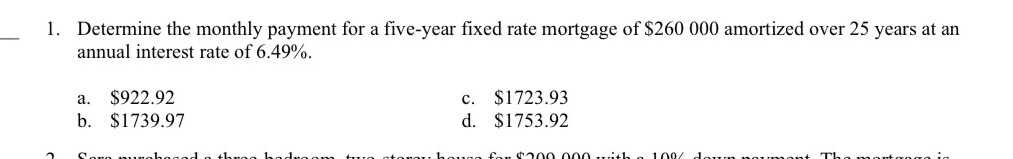### Still have math questions?

Arithmetic
Question1. Determine the monthly payment for a five-year fixed rate mortgage of $$\ 260000$$ amortized over $$25$$ years at an annual interest rate of $$6.49 \%$$ .

a. $$\ 922.92$$       c. $$\ 1723.93$$

b. $$\ 1739.97$$    d. $$\ 1753.92$$